# Coordinate

Determine missing coordinate of the point M [x, 120] of the graph of the function f bv rule: y = 5x

x =  2.97

### Step-by-step explanation: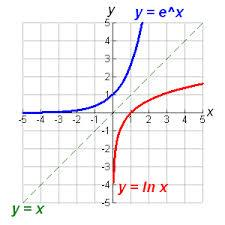Did you find an error or inaccuracy? Feel free to write us. Thank you!## Related math problems and questions:

• LogarithmDetermine the number whose decimal logarithm is -3.8.
• Exponential equationSolve exponential equation (in real numbers): 98x-2=9
• A Cartesian framework1. In a Cartesian framework, the functions f and g we know that: the function (f) is defined by f (x) = 2x ^ 2, the function (g) is defined by g (x) = x + 3, the point (O) is the origin of the reference, point (C) is the point of intersection of the graph
• Function 3Function f(x)=a(x-r)(x-s) the graph of the function has x- intercept at (-4, 0) and (2, 0) and passes through the point (-2,-8). Find constant a, r, s.
• Exponential equationIn the set R solve the equation: ?
• CrystalThe crystal grows every month 1.2 permille of its mass. For how many months to grow a crystal from weight 177 g to 384 g?
• Exponential equationSolve for x: (4^x):0,5=2/64.
• Exponential equationFind x, if 625 ^ x = 5 The equation is exponential because the unknown is in the exponential power of 625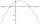It is given a quadratic function y = -4x2+5x+c with unknown coefficient c. Determine the smallest integer c for which the graph of f intersects the x-axis at two different points.
• Logif log4 x+ log8 x = log_b x, what is b?
• Sum-log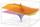The sum of two numbers is 32, the sum of their logarithms (base 10) is 2.2. Determine these numbers.
• Car valueThe car loses value 15% every year. Determine a time (in years) when its price will halve.
• Exponential equationDetermine the value of having y in the expression (3^y): (4^-1)=36. Unknown y is a natural number greater than zero.
• The city 3The city has 22,000 residents. How long it is expected to have 25,000 residents if the average annual population growth is 1.4%?
• SequenceCalculate what member of the sequence specified by (5n-2+15) has value 20.
• Half life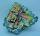Determine the half life of bismuth, when bismuth weight from the original weight of 32 g was only 2 grams in 242 minutes.
• Logarithmic equation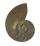Solve equation: log13(7x + 12) = 0